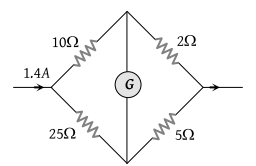NEET Questions Solved

PMT - 1989

In the circuit shown in the figure, the current flowing in 2 Ω resistance(1) 1.4 A

(2) 1.2 A

(3) 0.4 A

(4) 1.0 A

(4) Current through 2Ω $=1.4\left\{\frac{\left(25+5\right)}{\left(10+2\right)+\left(25+5\right)}\right\}=1A$

Difficulty Level:

• 18%
• 26%
• 24%
• 34%